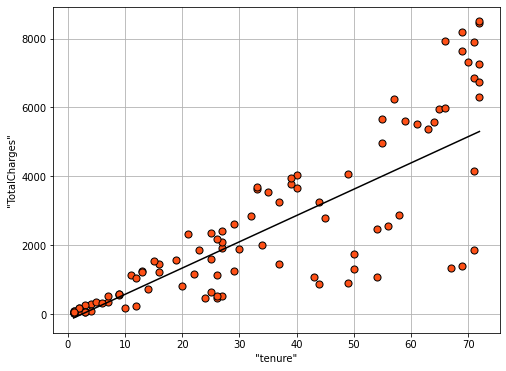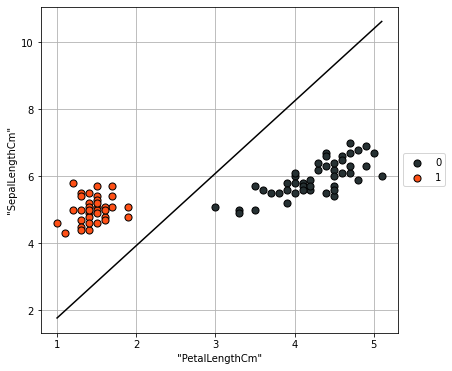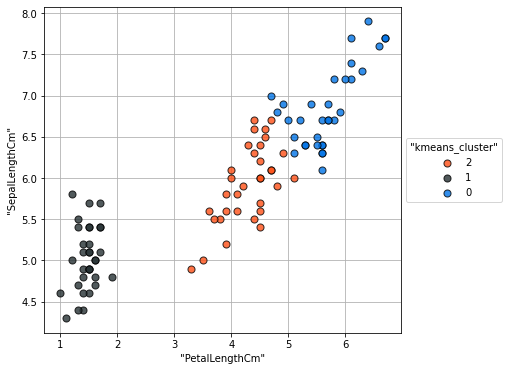### VerticaPy

Python API for Vertica Data Science at Scale

# Introduction to Machine Learning¶

One of the last stages of the data science life cycle is the Data Modeling. Machine learning algorithms are a set of statistical techniques that build mathematical models from training data. These algorithms come in two types:

• Supervised : these algorithms are used when we want to predict a response column.
• Unsupervised : these algorithms are used when we want to detect anomalies or when we want to segment the data. No response column is needed.

# Supervised Learning¶

Supervised Learning techniques map an input to an output based on some example dataset. This type of learning consists of two main types:

• Regression : The Response is numerical (Linear Regression, SVM Regression, RF Regression...)
• Classification : The Response is categorical (Gradient Boosting, Naive Bayes, Logistic Regression...)

For example, predicting the total charges of a Telco customer using their tenure would be a type of regression. The following code is drawing a linear regression using the 'TotalCharges' as a function of the 'tenure' in the Telco Churn Dataset.

In :
```import verticapy as vp

from verticapy.learn.linear_model import LinearRegression
model = LinearRegression("LR_churn")
model.fit(churn, ["tenure"], "TotalCharges")
model.plot()
```
Out:
`<AxesSubplot:xlabel='"tenure"', ylabel='"TotalCharges"'>`In contrast, when we have to predict a categorical column, we're dealing with classification.

In the following example, we use a Linear Support Vector Classification (SVC) to predict the species of a flower based on its petal and sepal lengths.

In :
```from verticapy.datasets import load_iris
iris.one_hot_encode()

from verticapy.learn.svm import LinearSVC
model = LinearSVC("svc_setosa_iris")
model.drop()
model.fit(iris, ["PetalLengthCm", "SepalLengthCm"], "Species_Iris-setosa")
model.plot()
```
Out:
`<AxesSubplot:xlabel='"PetalLengthCm"', ylabel='"SepalLengthCm"'>`When we have more than two categories, we use the expression 'Multiclass Classification' instead of 'Classification'.

# Unsupervised Learning¶

These algorithms are to used to segment the data (k-means, DBSCAN, etc.) or to detect anomalies (Local Outlier Factor, Z-Score Techniques...). In particular, they're useful for finding patterns in data without labels. For example, let's use a k-means algorithm to create different clusters on the Iris dataset. Each cluster will represent a flower's species.

In :
```from verticapy.learn.cluster import KMeans
model = KMeans("KMeans_iris", n_cluster = 3)
model.fit(iris, ["PetalLengthCm", "SepalLengthCm"])
model.plot()
```
Out:
`<AxesSubplot:xlabel='"PetalLengthCm"', ylabel='"SepalLengthCm"'>`In this section, we went over a few of the many ML algorithms available in VerticaPy. In the next lesson, we'll cover creating a regression model.